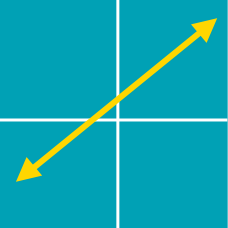Algebra

Linear Equations and Lines Warmup

A line passes through the point $(1,3).$ It also passes through exactly 1 of the following points: $(0,0), (6,6), (10,10), (3,9), (28,24).$ What is the slope of the line?

What is the equation of a line that passes through the points $(3,4)$ and $(a,b)$, assuming $a\neq3$ and $b\neq4$?

The line $L$ passes through the point $(4,4)$, has slope $m$ such that $m<-1$, and has $x$-intercept $a$ and $y$-intercept $b$ such that $a+b=18$.

What is the value of $b$?

There is one point $(a,b)$ such that the line $(k-3)x + (2k+1)y = 14$ passes through $(a,b)$ for all real values of $k$.

Find $a+b$.

Two distinct points $(a,b)$ and $(b,c)$ lie on the line $y=-x+5$ What is the value of $a-c$?

×